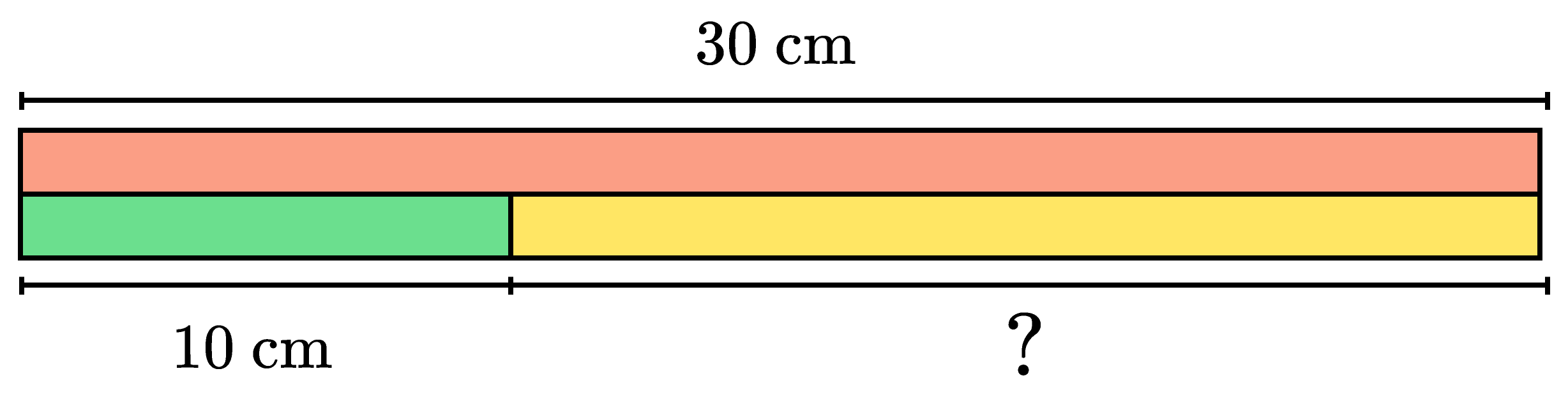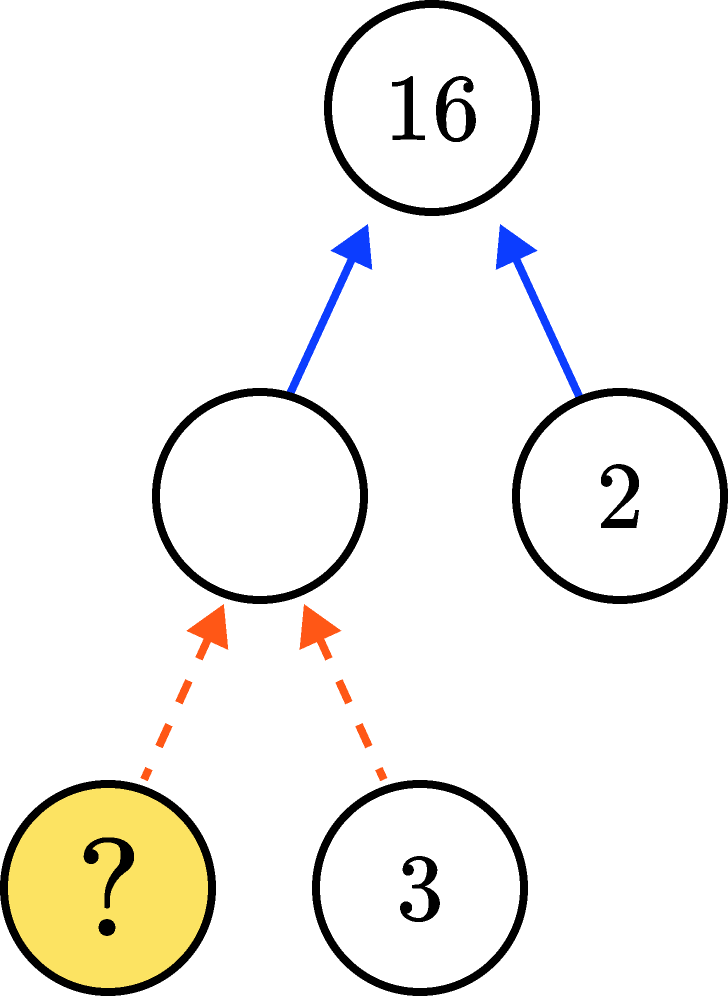### Pre-Algebra

Welcome to Pre-Algebra! This course can serve as a first introduction to algebra, a refresher for someone who hasn't studied it in a while, or another perspective for someone learning the fundamentals of algebra.

There's a common perception of algebra as "putting letters to arithmetic" as in the equation

$10 + x = 30,$

and while letters do get introduced, the essence of algebra is that instead of just doing arithmetic you are answering questions about arithmetic. Letters don't necessarily need to be used!

This course includes thinking with words and thinking with visuals. We'll still get to using letters, but we'll ground every step in intuitive understanding.

Let's get started!

# Thinking With Words and VisualsThe length of the entire bar is $30$ centimeters. The shorter portion marked green is $10$ centimeters. What is the length of the yellow portion with the question mark?

# Thinking With Words and VisualsDashed lines mean add, and solid lines mean multiply.

For example, two dashed lines point from $?$ and $3,$ so we add those numbers together to fill in the circle they point at.

What number must go in the yellow marked circle?

# Thinking With Words and Visuals

You can move the "First game" slider to help solve this problem!

Zofia bought two video games. Their prices had a difference of $\15,$ and she spent $\45$ total. How much did the cheaper of the two games cost?

# Thinking With Words and Visuals

Visuals and words can only go so far (otherwise, the symbols of algebra wouldn't have been invented in the first place!).

Suppose we change one of the numbers on the last problem:

Zofia bought two video games. They had a difference in cost of ${\15},$ and she spent ${\40}$ total. How much did the cheaper of the two games cost?

The games will now cost ${\27.50}$ and $\12.50$ (check it, if you want!), but these are hard numbers to come up with using a purely visual approach. Using and applying symbols make this type of problem easier to solve, and we'll try a few examples in the next quiz.

We'll still keep using intuition every step of the way!

×